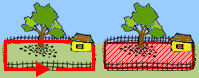# Perimeter and Area ExamplesDirections: Read each question below. Click once in an ANSWER BOX and type in your answer; then click ENTER. Your answers should be given as whole numbers greater than zero. After you click ENTER, a message will appear in the RESULTS BOX to indicate whether your answer is correct or incorrect. To start over, click CLEAR.

 1.Find the perimeter of a triangle with sides measuring 6 centimeters, 8 centimeters and 9 centimeters.  ANSWER BOX:=  cm  RESULTS BOX:
 2.A rectangle has a length of 9 inches and a width of 4 inches. Find the perimeter.  ANSWER BOX:=  in  RESULTS BOX:
 3.Find the perimeter of a regular octagon, with each side measuring 7 meters.  ANSWER BOX:=  m  RESULTS BOX:
 4.Find the area of a square with each side measuring 5 inches.  ANSWER BOX:=  in2  RESULTS BOX:
 5.A rectangular yard has a length of 16 meters and a width of 3 meters. Find the area.  ANSWER BOX:=  m2  RESULTS BOX:
 6.A rectangle has an area of 56 square feet and a width of 4 feet. What is the length?  ANSWER BOX:=  ft  RESULTS BOX:
 7.Find the area of a parallelogram with a base of 9 centimeters and a height of 5 centimeters.  ANSWER BOX:=  cm2  RESULTS BOX:
 8.The area of a parallelogram is 24 square inches and the base is 4 inches. Find the height.  ANSWER BOX:=  in  RESULTS BOX:
 9.Find the area of a triangle with a base of 7 meters and a height of 4 meters.  ANSWER BOX:=  m2  RESULTS BOX:
 10.Find the area of a trapezoid with bases of 9 inches and 13 inches, and a height of 4 inches.  ANSWER BOX:=  in2  RESULTS BOX: